Radmanović, K., Đukić, I., Merhar, M., Šafran, B., Jug, M., and Beljo Lučić, R. B. (2018). "Longitudinal and tangential coefficients of chip compression in orthogonal wood cutting," BioRes. 13(4), 7998-8011.

#### Abstract

The complexity of the cutting zone makes it impossible to establish a reliable, simple, and comprehensive mathematical model for orthogonal wood cutting. Thus far, the science of cutting materials have used only approximate and simplified engineering theories that attempt to explain the mechanisms of chip shaping. The research of wood processing by chip separation is mostly based on models developed for metal processing. By applying Astakhov’s model to orthogonal wood cutting, the total cutting power is divided into four components, and each cutting force component can be theoretically related to the coefficients of chip compression.  In the present study, longitudinal, and tangential coefficients of chip compression were determined experimentally during orthogonal cutting of wood in the longitudinal (0 – 90) direction depending on wood moisture content (MC) and cutting speed. The direction of the fibers, cutting speed, and MC influenced the ​​ coefficient of chip compression. The MC of wood above the fiber saturation point significantly changed the impact of the cutting speed on the tangential coefficient of chip compression. In order to explain and better understand the obtained results of chip compression coefficients, the modulus of elasticity (MOE) of wood samples with different MC was determined. The MOE was calculated from the compression tests on small specimens in tangential and longitudinal direction.

Longitudinal and Tangential Coefficients of Chip Compression in Orthogonal Wood Cutting

Kristijan Radmanović, Igor Đukić, Miran Merhar, Branimir Šafran, Matija Jug, and Ružica Beljo Lučić

The complexity of the cutting zone makes it impossible to establish a reliable, simple, and comprehensive mathematical model for orthogonal wood cutting. Thus far, the science of cutting materials have used only approximate and simplified engineering theories that attempt to explain the mechanisms of chip shaping. The research of wood processing by chip separation is mostly based on models developed for metal processing. By applying Astakhov’s model to orthogonal wood cutting, the total cutting power is divided into four components, and each cutting force component can be theoretically related to the coefficients of chip compression. In the present study, longitudinal, and tangential coefficients of chip compression were determined experimentally during orthogonal cutting of wood in the longitudinal (0 – 90) direction depending on wood moisture content (MC) and cutting speed. The direction of the fibers, cutting speed, and MC influenced the ​​ coefficient of chip compression. The MC of wood above the fiber saturation point significantly changed the impact of the cutting speed on the tangential coefficient of chip compression. In order to explain and better understand the obtained results of chip compression coefficients, the modulus of elasticity (MOE) of wood samples with different MC was determined. The MOE was calculated from the compression tests on small specimens in tangential and longitudinal direction.

Keywords: Orthogonal wood cutting; Chip compressionLongitudinal and tangential coefficients; Cutting speed; Modulus of elasticity; Wood moisture content

Contact information: University of Zagreb, Faculty of Forestry, Department of Process Engineering, Svetošimunska25, 10 000 Zagreb, Croatia; *Corresponding author: kradmanovic@sumfak.hr

INTRODUCTION

Orthogonal cutting occurs when the cutting tool of specific geometry penetrates the material of the workpiece, whereby the edge of the blade is perpendicular to the direction of the tool’s motion. A thorough knowledge of the process is the main condition for the construction of tools and machines that will conceptually result in providing optimum production and achieving high quality products, in conjunction with the increasing demands for accuracy and quality of the treated surface. The first systematic research of cutting forces in woodworking began in the 1950s with the studies of Kivimaa (1952), Franz (1958), and McKenzie (1961). These authors used simple mechanical patterns for determining the cutting forces and generally did not describe the physical background of the process itself. Empirical models are used often in research on wood – processing by chip separation. They can be useful in practice, but are largely limited to specific processing conditions, and outside of these conditions they are usually not valid and do not give a physical explanation of the process of particle separation. Naylor et al.(2012) determined empirical expressions for the cutting force depending on mechanical characteristics of wood, cutting depth, and MC.

The commonly used empirical model for determining the cutting force via the cutting resistance (Kr) is shown in Eq. 1 (Lisičan1996),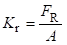(1)

where Kr is the resistance of cutting (MPa), Fis the cutting force (N), and is the cross-sectional area of chips (m2).

The specific resistance is affected by a large number of parameters such as wood species, chip thickness, rake and clearance angle, friction, cutting speed, and wood MC. The measured values of the specific cutting resistance within the same wood species, and even within the same board, can differ by up to 25% (Goglia 1994). The specific cutting resistance is determined by the so-called reference specific cutting resistance (Kφ). The reference for specific cutting resistance is determined experimentally under strictly defined and controlled conditions that consider the direction of wood fibers. All other influencing parameters are known, and their values in measuring the specific cutting resistance are called reference values of influencing parameters.

In metal processing, a series of models for determining the cutting force have been applied in which the complex state of stress and deformation in the cutting zone tends to correlate with values that can be experimentally determined in real conditions (Arrazola et al. 2013). In the orthogonal metal cutting model, derived from the Ernst-Merchant theory, Atkins (2003) includes the fracture mechanics and specific energy needed to create a new surface. According to Atkins, the force applied in the orthogonal cutting system is the result of summing three components: the power needed for the plastic deformation of the chip, the power needed to overcome the friction force on the front side of the tool, and the power to create a new surface. Atkins outlines good correlation between theoretical and experimental results in metal cutting, and also states that the model can be applied to the process of wood cutting providing that continuous chips are produced. By incorporating fracture mechanics into the model, the singularity of solutions in the area of ​​maximum stress, namely the very tip of the blade, is introduced.

Astakhov and Xiao (2008) applied the energy approach for determining the cutting force in metal processing and explained the model at the level of the basic laws of physics, and a large part of this research is based on previous studies of Zorev (1966). According to Astakhov and Xiao (2008), the total cutting force of metal is the result of summing the power required for the plastic chip deformation (Ppd), the power required to overcome the friction on the front side of the tool (P(fr)f), the power required to overcome the friction on the back side of the tool (P(fr)b), and the power required to create a new surface (Pns). The model developed by Astakhov and Xiao (2008) is based on using continuum mechanics as a physical background for connecting the characteristics of the workpiece material and technological parameters of processing. The results of the model show a good correlation with experimental data in metal processing.

It is important to stress the known fact that the metal is a homogeneous material, whereas wood is anisotropic. Because of the different mechanical properties, the ranges of cutting speeds in woodworking are significantly higher than in metal working, and cutter parameters are also different. Nevertheless, in some conditions the Astakhov model could be applied for analyses of the cutting process of wood.

By applying the Astakhov model on the process of orthogonal wood cutting, provided that continuous chips are produced, each component of the cutting force can be connected with the coefficients of chip compression, as factors that could be measured relatively easily in practice and be the value of the total stress occurring in the cutting zone. Figure 1 shows the process of orthogonal cutting of wood in the longitudinal direction that can be observed as the process of transformation of a part of workpiece of dimensions (x0y0) into chip dimensions (x1y1). By comparing the deformed and undeformed state, it can be concluded that during the cutting process, chip length contraction (x0) and height contraction (y0) (xx1yy1) occur. It is assumed that length contraction (x0) occurs due to compression stress parallel to the fibers in longitudinal direction, and height contraction (y0) occurs due to compression stress perpendicular to the fibers in tangential direction.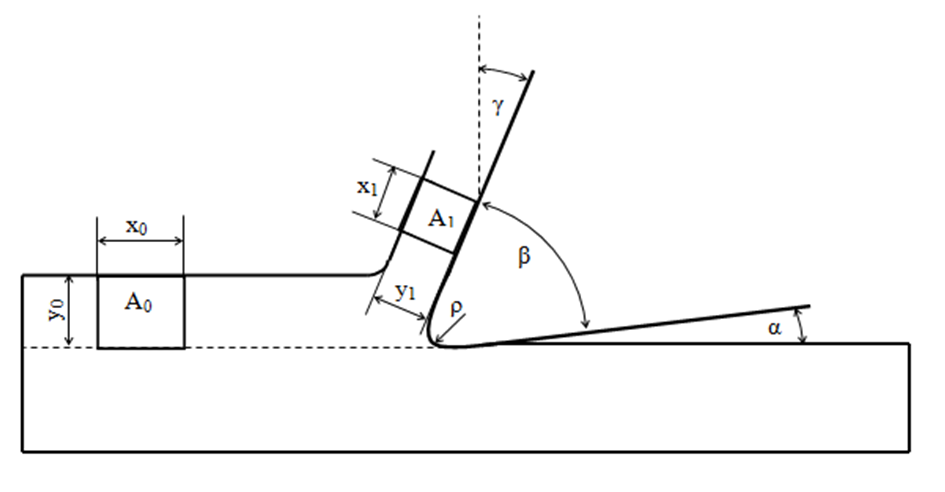Fig. 1. Process of plastic chip deformation in orthogonal wood cutting: x0 – length of uncut chip, y0 – thickness of uncut chip, x1 – length of cut chip, y1 – thickness of cut chip, A0 – surface of uncut chip, A1 – surface of cut chip, α -clearance angle, β – wedge angle, γ– rake angle, ρ – radius of the cutting edge

Equation 2 represents the longitudinal coefficient and Eq. 3 represents the tangential coefficient of the chip compression. In Eqs. 2 and 3, the deformed and undeformed state of cut wood are compared,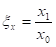(2)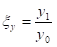. (3)

where ξx is the the coefficient of chip compression in longitudinal direction, x1 is the length of the deformed chip, x0 is the length of the undeformed chip, ξy is the coefficient of chip compression in tangential direction, y1 is the height of the deformed chip, and y0 is the height of the undeformed chip.

The surface coefficient of compression can be determined from Eq. 4,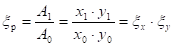, (4)

where ξis the ratio of the surface of the deformed (A1) and the undeformed state (A0). In processing isotropic materials, it is necessary to meet the condition of constant surface (Astakhov and Xiao 2008), ξp = 1, i.e. ξx = ξy-1, while in processing non-isotropic materials, the following applies: ξx ≠ ξy-1 and ξp ≠ 1. Applying the continuity equation to the process of orthogonal wood cutting (Radmanović 2015), it follows that the ratio of the wood density of cut and uncut chip is inversely proportional to the surface coefficient of compression as shown in Eq. 5,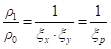, (5)

where ρis the density of the cut chip (kg/m3), and ρis the density of the uncut chip (kg/m3).

The aim of this paper was to determine the longitudinal and tangential coefficients of chip compression in the orthogonal cutting of wood in the longitudinal direction depending on the cutting speed and wood MC and to determine the MOE on compressive stress depending on the wood MC.

EXPERIMENTAL

Experimental research was completed in three phases. In the first phase, the samples were selected and prepared. In the second phase, the MOE of the samples was evaluated parallel and perpendicular to the fibers in the tangential direction. In the third phase, the coefficients of longitudinal and tangential chip compression were determined in orthogonal cutting of samples. The measurement of the MOE of samples was completed in the accredited Laboratory for Wood in Construction of the Faculty of Forestry of Zagreb University (Zagreb, Croatia), and the measurement of coefficients of chip compression in orthogonal cutting of samples was completed at the Department of Wood Science and Technology of the Biotechnical Faculty in Ljubljana (Ljubljana, Slovenia).

Materials

The research was carried out on pine samples (Pinus sylvestris L.) with no visible wood defects. Observation based on volume showed an approximately equal share of early and late wood.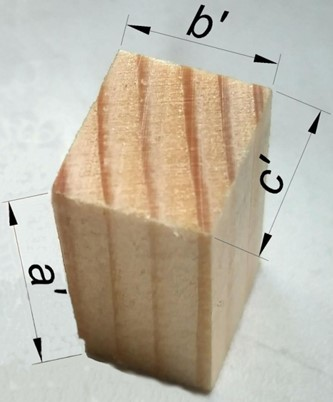Fig. 2. Pine sample used for testing compressive characteristics in longitudinal and tangential direction. a’ – sample dimension in longitudinal direction, b’ – sample dimension in tangential direction and c’ – sample dimension in radial direction.

For determining the MOE of pine, depending on wood MC, samples with the following dimensions were prepared: a’ = 1.00 ± 0.01 cm, b’ = 2.00 ± 0.01 cm, c’ = 1.25 ± 0.01 cm as shown in Fig. 2. They were conditioned at water content (MC = 0, 10, 14, 30, and 45 %) by standardized methods. It is important to mention that the sample dimensions are not in accordance with the standardized values of samples for testing compressive strength. It is known that the mechanical characteristics of wood depend on sample dimensions and wood content, respectively (Horvat and Krpan 1967). Thus, efforts were made to determine the elasticity of the material on samples of dimensions as small as possible, which could be accurately tested on the testing machine, in order to get representative values of the MOE for the purpose of this study.

For determining the dependence of the coefficient of chip compression on wood MC, 20 samples with the following dimensions were prepared (= 12.52 ± 0.04 cm, b = 5.82 ± 0.02 cm, and c = 1.25 ± 0.01 cm). They were conditioned to MC = 10, 14, 30, and 45 %. Lines (0.3 ÷ 0.5 mm thick) were drawn on the samples by a waterproof marker pen (Fig.3) to make the coefficient of chip compression easier to read.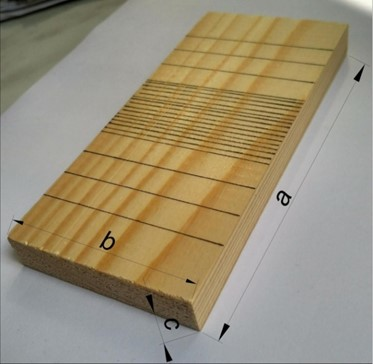Fig. 3. Sample illustration. a – sample dimension in longitudinal direction (sample length), b – sample dimension in tangential direction (sample width), c – sample dimension in radial direction (sample thickness)

Methods

Determination of modulus of elasticity

MOE was determined in the longitudinal and tangential directions. MOE was tested on an autograph machine (Shimadzu Autograph AG-X, Maryland, USA) equipped with dynamometer with a nominal force of 10,000 N. The testing was completed by using the software Trapezium X (Shimadzu, Maryland, USA). The MOE was tested depending on the sample MC, where MC was approximately 10, 14, 30, and 45 %. The experiment was repeated 3 times under the same conditions. Data on the measured force and shear were further processed using a software package (Sigmaplotversion10.0, Systat Software Inc, San Jose, USA).

Compression stress in the longitudinal direction was determined according to Eq. 6 and the relative deformation was determined according to Eq. 7,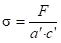, (6)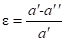, (7)

where σ is the stress (MPa), F is the force (N), a‘ is the the initial sample length (cm), a” is the final sample length (cm), and c‘ is the the initial sample thickness (Fig. 4).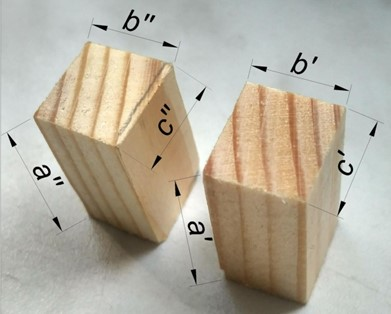Fig. 4. Pine sample before and after determining the modulus of elasticity parallel to fibers. a’ – sample dimension in longitudinal direction before testing, b’ – sample dimension in tangential direction before testing, c’ – sample dimension in radial direction before testing, a’’ – sample dimension in longitudinal direction after testing, b’’ – sample dimension in tangential direction after testing, c’’ – sample dimension in radial direction after testing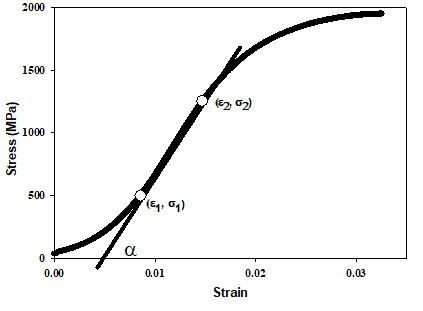Fig. 5. Diagram of deformation dependence on compression strength parallel to fibers

In the diagram of deformation stresses, in the area of the curve (Fig. 5) where the stress and deformation ratio is linear, the values of stress and deformation can be interpreted in two close points of (ε 1, σ1)and ( ε2, σ2). Thus, the MOE is determined according to Eq. 8,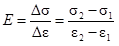(8)

where σ1 and σare the two values of stress in the elastic area (MPa), and ε1 and εare the two values of strain in the elastic area.

Determination of coefficients of chip compression

Orthogonal cutting in the longitudinal direction (0° to 90°) of pine samples was 125 mm × 12 mm, and the experimental setup was used as shown in Fig. 6. The basis of this system consisted of a movable iron board with the sample attached. Cutting depth was 0.3 mm, and it was determined using a screw and accuracy comparator (Model2114-5F, Insize, Zamudio, Spain) of 0.01 mm. The shear board was connected to a drive electric motor that can achieve a speed of up to 5 m/s. A blade consisting of high-speed cutting steel (HSS) was used for cutting with the wedge angle of 30°, and it was pre-sharpened. The rake angle of the blade was adjusted in order to get continuous type II chips. According to Koch (1966), for the selected MC an angle of 10° is suitable. The radius of the cutting edge was 2 μm.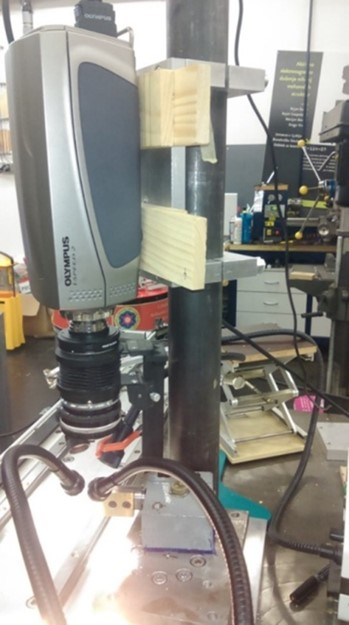Fig. 6. Experimental setup of camera with lenses mounted above tool blade with halogen light

To determine the coefficients of chip compression, the cutting process was recorded by a quick camera Olympus I – Speed 2, which was mounted laterally with respect to the tool blade, and the cutting process was recorded at 1000 and 1400 frames/s. With lenses mounted on the camera, the visual field of 5.6 mm × 4.2 mm was achieved, and the halogen light that focused on the measurement area was sufficient for illuminating such a small surface. The camera’s recordings were later processed in a software package (I -SPEED, Olympus, Tokyo, Japan), where the coefficients of chip compression were determined. During the experiment, the values of MC were varied in the range of 10, 14, 30, and 45 %, and the cutting speed (vc) was in the range of 0.5, 1, 1.5, 2, and 3 m/s. A cutting depth of 0.3 mm was used for the calibration of the image. Figures 7a and 7b show an example of the dimensions of uncut and cut chips. The dimensions of the uncut chips were measured before the deformation process started, and when the tool blade was far enough not to cause compression in the marked part of the workpiece. The dimensions of the cut chip were measured at the moment when the marked part of the chip was not in contact with the front surface of the tool. Figure 7b shows the angle closed by the chip length (x1) and height (y1) other than 90°. It is assumed that the angle changed during the deformation process due to tangential stress. The experimental study showed that under the set processing conditions, the angle deformations were remarkably low and could be neglected. The coefficients of chip compression were determined based on the ratio of dimensions of cut and uncut chips. Cutting was repeated 3 times under the same conditions. The coefficients of chip compression were measured 10 times under the same cutting conditions, and then the mean value and the respective standard deviation were determined.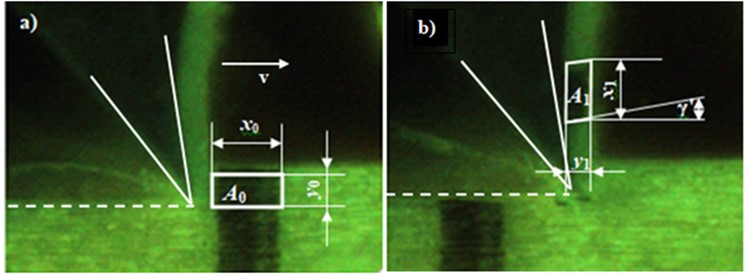Fig. 7. Process of plastic chip deformation in orthogonal wood cutting: a) underformed state, b) deformed state, x0 – length of uncut chip, y0 – thickness of uncut chip, x1 – length of cut chip, y1 – thickness of cut chip, A0 – surface of uncut chip, A1 – surface of cut chipγ’ – shear angle

RESULTS AND DISCUSSION

Results of Measurement of Modulus of Elasticity

The MOE of the samples was parallel to the fibers and perpendicular to the fibers in the tangential direction depending on the sample moisture content. Under these conditions, the experiment was repeated three times, and then the mean value and standard deviation were determined. The results of measurement are shown in Table 1.

Table 1. Measurement Results of MOE Obtained from Compression Tests in the Longitudinal and Tangential Directions Depending on the MC of Samples with Respective Standard Deviations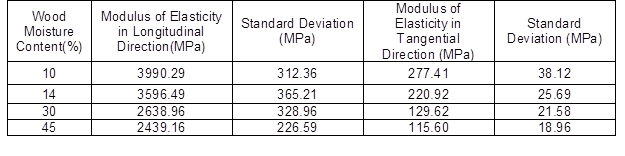Table 1 shows that with the increase of MC, the mean value of both moduli of elasticity decreased, which is consistent with Požgaj et al. (1993). Elastic deformation was inversely proportional to the modulus of elasticity (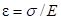). With an increase in the MC, the elastic deformation also increases. Figure 8 shows the ratio between the MOE in the longitudinal and tangential direction for MC = 10, 14, 30, and 45 % with the respective standard deviation. It was evident in the diagram that with the increase of the MC, this ratio increases. This means that with the increase of the MC, the MOE in the longitudinal direction decreased more, becoming lower than the MOE in the tangential direction. Moreover, with the increase of the MC, the elastic deformation increased faster in the tangential direction compared with elastic deformation in the longitudinal direction.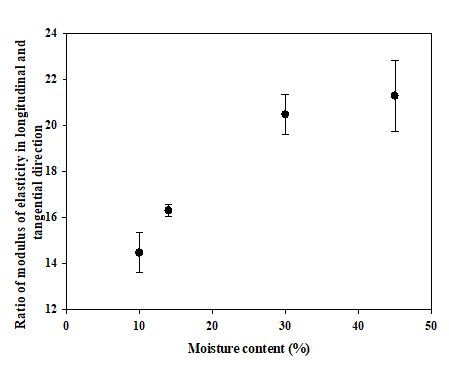Fig. 8. Ratio of modulus of elasticity in longitudinal and tangential for MC = 10, 14, 30, and 45 % with the respective standard deviation

Results of Measurement of Chip Compression Coefficient

The coefficients of chip compression were determined from the camera recording in accordance with the procedure described in the section Determination of coefficients of chip compression. Table 2 shows the results of measurement of the compression coefficient with the respective standard deviation on the basis of 10 measurements under the same conditions. Figure 8 shows the mean value dependence of the longitudinal coefficient of chip compression on the cutting speed at different MC. It was observed from the diagram of dependence that the mean value of the longitudinal coefficient of chip compression decreased with the increase of the cutting speed for all tested values of MC. The decrease in the mean value of the coefficient of chip compression was higher for the cutting speed from 0.5 m/s to 1.5 m/s, while the decrease in the value was considerably lower for the speed from 1.5 m/s to 3 m/s. For MC of up to 30 %, the mean value of the longitudinal coefficient of chip compression decreased for all cutting speeds used. For wood with 45 % MC, at a cutting speed from 1.5 m/s to 3 m/s, the longitudinal coefficient of chip compression increased. The results of the measurement of elastic properties of wood samples with MC higher than the fiber saturation point indicated an increase of elastic deformations in the longitudinal direction, which can be caused by higher elastic material recovery of the chip, and hence also of the increase of the compression coefficient in cutting wood with high MC.

Table 2. Results of Measurement of Chip Compression Coefficients Depending on Sample Moisture Content and Cutting Speed with Respective Standard Deviations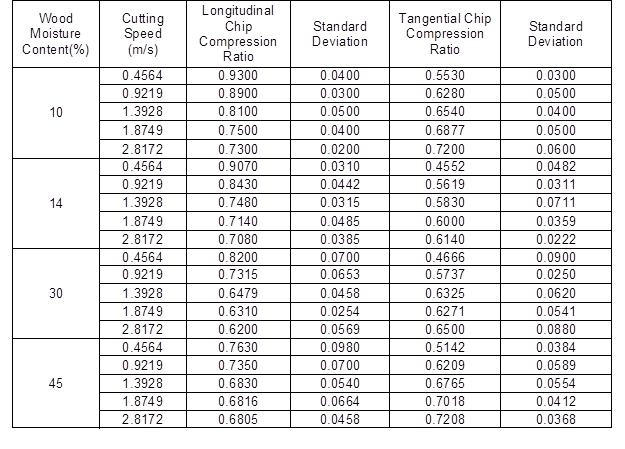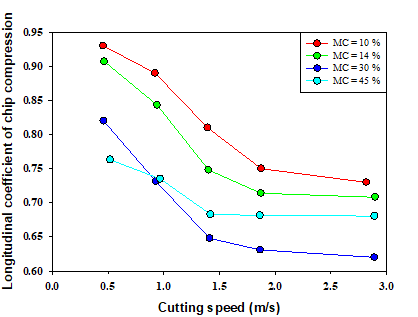Fig. 9. Diagram of mean value dependence of longitudinal coefficient of chip compression on cutting speed for MC = 10, 14, 30, and 45 %

Figure 10 shows the diagram of mean value dependence of the tangential coefficient of chip compression on cutting speed at different MC. With the increase of the cutting speed, the mean value of the tangential coefficient of chip compression increased. This change was most prominent during the change of the cutting speed from 0.5 m/s to 1 m/s, for all tested MC. For the cutting speed range from 1.5 m/s to 3 m/s, the change of the mean value of the tangential coefficient of chip compression was considerably lower. Astakhov and Xiao (2008) states that in processing metal, the tangential coefficient of compression increases with the increase of the cutting speed. At a certain cutting speed, it reaches its maximum, and after that it decreases, while at higher speeds, it becomes constant. According to Astakhov and Xiao (2008), the cutting speed which has the highest coefficient of tangential compression, depends on the material of the workpiece and processing parameters.

The tangential coefficient of chip compression measured in cutting wood samples with MC of 30 and 45% was higher than the tangential coefficient measured in samples with MC lower than the fiber saturation point. This was due to the higher elastic recovery of the material decreasing due to lower MOE, which decreased considerably with the increase of MC in the tangential direction.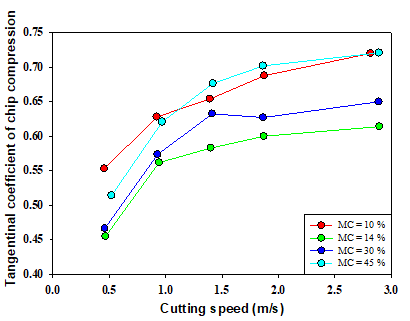Fig. 10. Diagram of mean value dependence of tangential coefficient of chip compression on cutting speed for MC = 10, 14, 30, and 45 %

Based on Eqs. 4 and 5, the surface coefficients of chip compression and density ratio between the cut and uncut chip were determined. Figure 11 presents the diagram of the mean value dependence of the surface coefficient of chip compression, and the density ratio of cut and uncut chips on the cutting speed at different MC. With the increase of the cutting speed up to 1 m/s, the mean value of the surface coefficient of chip compression increased for all tested wood MC. With further increase of the cutting speed, the value of the surface coefficient decreased for all tested MC, except for MC = 45%. The behavior of the density ratio of cut and uncut chips was reciprocal to the behavior of the surface coefficient of chip compression, which can be concluded by comparing Eqs.3 and 4. From Fig. 11, it can be concluded that the change in the surface coefficient of the chip compression depended on the cutting speed fitting well in the Astakhov’s model in the metal processing for all wood samples except those with MC of 45 %. In processing samples with MC = 10, 14, and 30 %, the surface coefficient of the chip compression increased with the increase of the cutting speed, and it reached its maximum at the cutting speed of 1 m/s, after which, it decreased and at higher speeds, it became constant. On the contrary, the surface coefficient of the chip compression increased with the increase of the chip cutting speed in processing samples with a MC of 45%, and it did not fit into the Astakhov’s model.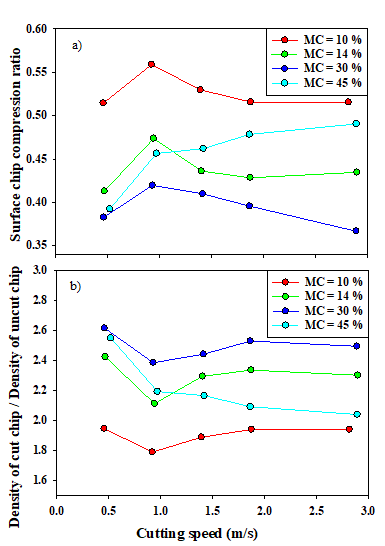Fig. 11. Diagram of mean value dependence of surface coefficient of chip compression (a), and density ratio of cut and uncut chip on cutting speed for MC =10, 14, 30, and 45 % (b)

CONCLUSIONS

1. The results of this research have shown that, with the increase of wood MC, the MOE of wood decreases, i.e. the elastic deformation is higher, which is in line with the results of other studies. The change of the modulus of elasticity of wood with the increase of moisture content is higher in the tangential direction than in the longitudinal direction.
2. The longitudinal coefficient of chip compression decreases, while the tangential coefficient of compression increases with the increase of the cutting speed for the samples with MC of 30% and 45%.
3. In cutting the samples with wood MC of 30% and 45%, the coefficients of chip compression increase with the increase of speed. The highest tangential coefficients of chip compression were measured on samples with the highest moisture content. It can be concluded from the obtained results that, in cutting samples with wood MC of 30% and 45%, the change of elastic properties of wood has a higher influence on the tangential coefficient of compression than the change of other mechanical properties of wood, while the change of the surface coefficient of chip compression depending on the cutting speed does not fit into the Astakhov’s model in metal cutting.
4. The results of the research have shown that although wood is an anisotropic material, under certain processing conditions the Astakhov’s model, which is used in analyses of processing of metal as an isotropic material, can be adapted and used for the analysis of the wood cutting process.
5. The coefficients of compression (as factors that could be easily determined in practice) represent the link between the mechanical characteristics of the workpiece and the processing parameters. The results of research will contribute to building the model for calculation of cutting forces during woodworking.

REFERENCES CITED

Arrazola, P.J., Ozel, T., Umbrello, D., Davies, M., and Jawahir, I. S. (2013). “Recent advances in modeling of metal machining processes,” CIRP Annals – Manufacturing Technology62(2), 695-718.DOI: 10.1016/j.cirp.2013.05.006

Astakhov, V. P., and Xiao, X. (2008). ”A methodology for practical cutting force evaluation based on the energy spent in the cutting system,” Machining Science and Technology 12,325-347.DOI: 10.1080/10910340802306017

Astakhov, V. P. (1998). ”Metal Cutting Mechanics,” CRC Press, Boca Raton, FL, USA.

Atkins, A. G. (2003). ”Modeling metal cutting using modern ductile fracture mechanics: quantitative explanations for some longstanding problems,” International Journal of Mechanical Sciences 45(2), 373-396.DOI: 10.1016/S0020-7403(03)00040-7

Franz, N. C. (1958). An Analysis of the Wood-cutting Process, The University of Michigan Press, Ann Arbor, MI, USA.

Goglia, V. (1994). Strojevi I Alati za Obradu Drva (Woodworking machines and tools), Faculty of Forestry University of Zagreb, Zagreb, Croatia.

Horvat, I., and Krpan, J. (1967). Drvnoindustrijski Priručnik (Wood – processing manual), Tehnička knjiga Zagreb, Zagreb, Croatia.

Kivimaa, E. (1952). ”Die Schnittkraft in der Holzbearbeitung” (The cutting force in the woodworking), HolzRohWerk. 10: 94108

Lisičan, J. (1996). Teorija a Technika Spracovania Dreva (Theory and woodworking technology), MAT-CENTRUM, Zvolen, Slovakia.

McKenzie, P., Helnwein, J., Eberhardsteiner, H., and Mang, A. (2003). “A multi surface plasticity model for clear wood and its application to the finite element analysis of structural details,” Computational Mechanics 31(1-2), 204-218. DOI:10.1007/s00466-003-0423-6

McKenzie, W. M., (1961). Fundamental Analysis of the Wood-cutting Process, PhD thesis,University of Michigan, MI, USA.

Naylor, A., Hackney, P., Perera, N., and Clahr, E. (2012). “A predictive model for the cutting force in wood machining developed using mechanical properties,” BioResources 7(3), 2883-2894.DOI:10.15376/biores.7.3.2883-2894

Požgaj, A., Chovanec, D., Kurjatko, S., and Babiak, M. (1993). Štruktura a Vlastnosti Dreva (Structure and properties of wood), Prirodaa. s., Bratislava, Slovakia.

Radmanović, K. (2015). Utjecaj Mehaničkih Svojstava Drva na Snagu Potrebnu za Odvajanje Čestice pri Ortogonalnom Rezanju (The influence of mechanical properties of wood to the power required for separating chip in orthogonal cutting), PhD thesis, Faculty of Forestry University of Zagreb, Zagreb, Croatia.

Zorev, N. N. (1966). Metal Cutting Mechanics, Pergamon Press, New York .

Article submitted: February 2, 2018; Peer review completed: April 9, 2018; Revised version received: July 4, 2018; Accepted: July 5, 2018; Published: September 7, 2018.

DOI: 10.15376/biores.13.4.7998-8011